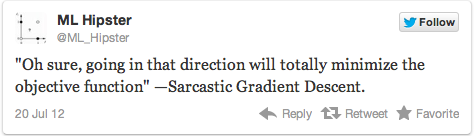# Stochastic Optimization

36-350
29 October 2014

### Agenda

• Why there's such a thing as too much data
• How to make noise our friend
• How to make slop our friend

$\newcommand{\Expect}{\mathbb{E}\left[ #1 \right]} \newcommand{\Var}{\mathrm{Var}\left[ #1 \right]} \newcommand{\ER}{f} \newcommand{\TrueR}{f_0} \newcommand{\ERM}{\hat{\theta}_n} \newcommand{\EH}{\widehat{\mathbf{H}}_n} \newcommand{\tr}{\mathrm{tr}\left( #1 \right)}$

### Problems with Big Data

• Typical statistical objective function, mean-squared error: $f(\theta) = \frac{1}{n}\sum_{i=1}^{n}{{\left( y_i - m(x_i,\theta)\right)}^2}$

• Getting a value of $$f$$ is $$O(n)$$, $$\nabla f$$ is $$O(np)$$, $$\mathbf{H}$$ is $$O(np^2)$$

• worse still if $$m$$ slows down with $$n$$
• Not bad when $$n=100$$ or even $$n=10^4$$, but if $$n={10}^9$$ or $$n={10}^{12}$$ we don't even know which way to move

### Problems with Big Data### Sampling, an Alternative to Sarcastic Gradient Descent

• Pick one data point $$I$$ at random (uniform on $$1:n$$)

• Loss there, $${\left( y_I - m(x_I,\theta)\right)}^2$$, is random, but

$\Expect{{\left( y_I - m(x_I,\theta)\right)}^2} = f(\theta)$

• Generally, if $$f(\theta) = n^{-1}\sum_{i=1}^{n}{f_i(\theta)}$$ and $$f_i$$ are well-behaved, $\begin{eqnarray*} \Expect{f_I(\theta)} & = & f(\theta)\\ \Expect{\nabla f_I(\theta)} & = & \nabla f(\theta)\\ \Expect{\nabla^2 f_I(\theta)} & = & \mathbf{H}(\theta) \end{eqnarray*}$

$$\therefore$$ Don't optimize with all the data, optimize with random samples

Draw lots of one-point samples, let their noise cancel out:

1. Start with initial guess $$\theta$$, learning rate $$\eta$$
2. While ((not too tired) and (making adequate progress))
• At $$t^{\mathrm{th}}$$ iteration, pick random $$I$$ uniformly
• Set $$\theta\leftarrow \theta - t^{-1}\eta\nabla f_I(\theta)$$
3. Return final $$\theta$$

Shrinking step-size by $$1/t$$ ensures noise in each gradient dies down

(Variants: put points in some random order, only check progress after going over each point once, adjust $$1/t$$ rate, average a couple of random data points (“mini-batch”), etc.)

stoch.grad.descent <- function(f,theta,df,max.iter=1e6,rate=1e-6) {
for (t in 1:max.iter) {
theta <- theta - (rate/t)*g
}
return(x)
}

stopifnot(require(numDeriv))
i <- sample(1:nrow(df),size=1)
noisy.f <- function(theta) { return(f(theta, data=df[i,])) }
}


### Stochastic Newton's Method

a.k.a. 2nd order stochastic gradient descent

1. Start with initial guess $$\theta$$
2. While ((not too tired) and (making adequate progress))
• At $$t^{\mathrm{th}}$$ iteration, pick uniformly-random $$I$$
• $$\theta \leftarrow \theta - t^{-1}\mathbf{H}_{I}^{-1}(\theta) \nabla f_{I}(\theta)$$
3. Return final $$\theta$$

+ all the Newton-ish tricks to avoid having to recompute the Hessian

• Pros:

• Each iteration is fast (and constant in $$n$$)
• Never need to hold all data in memory
• Does converge eventually
• Cons:

• Noise does reduce precision — more iterations to get within $$\epsilon$$ of optimum than non-stochastic GD or Newton

Often low computational cost to get within statistical error of the optimum

### How Precise?

• We're minimizing $$f$$ and aiming at $$\hat{\theta}$$

• $$f$$ is a function of the data, which are full of useless details

• e.g., $$f(\theta) = n^{-1}\sum_{i=1}^{n}{(y_i-m(x_i;\theta))^2}$$
• We hope there's some true $$f_0$$, with minimum $$\theta_0$$

• e.g., $$f_0(\theta) = \Expect{(Y-m(X;\theta))^2} = \int{(y-m(x;\theta))^2 p(x,y) dx dy}$$
• but we know $$f \neq f_0$$

• because we've only got a finite sample, which includes noise
• Past some point, getting a better $$\hat{\theta}$$ isn't helping is find $$\theta_0$$

(why push optimization to $$\pm {10}^{-6}$$ if $$f$$ only matches $$f_0$$ to $$\pm 1$$?)

### How Precise?

• An example
• true model: $$Y=X +$$ Gaussian noise with mean 0, s.d. 0.1, $$X$$ uniform between 0 and 1
• Try regression through the origin ($$Y=bX$$, $$b$$ unknown)
• We'll plot the population objective function, and a bunch of sample objective functions from different $$n=30$$ draws
f0 <- function(b) { 0.1^2+ (1/3)*(b-1)^2 }
f <- Vectorize(FUN=function(b,df) { mean((df$y - b*df$x)^2) }, vectorize.args="b")
simulate_df <- function(n) {
x <- runif(n)
y<-x+rnorm(n,0,0.1)
return(data.frame(x=x,y=y))
}


### How Precise?

curve(f0(b=x), from=0,to=2,)
replicate(100,curve(f(b=x,df=simulate_df(30)),